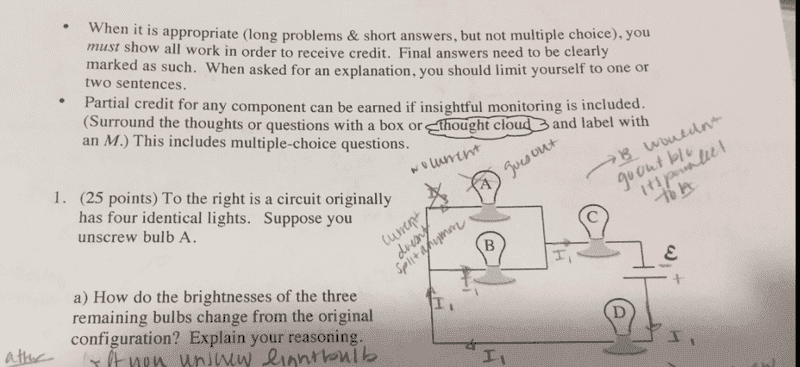# Removing a light bulb

## Homework Statement## Homework Equations

Ohm's Law, Equations for parallel and series combinations of resistors[/B]

## The Attempt at a Solution

Working this problem I obtain a solution that doesn't make sense, which is that the bulbs are dimmer.

If I assign 10 ohms to each bulb, I believe the total resistance would be:

$$R_D+R_C+\left(\frac{1}{R_A}+\frac{1}{R_B}\right)^{-1}=25\Omega$$

After removing A, it would just be 30 ohms.

Before: 25 ohms
After: 30 ohms

So now there's more resistance and less current? [/B]

Gold Member
2022 Award
So now there's more resistance and less current?
That's an unhelpfully vague statement. WHERE would there be more or less of something and what would the effect be?

TomHart
Less overall current - true. So figure out each bulb individually to see how much current - before and after.

Less overall current - true. So figure out each bulb individually to see how much current - before and after.

Right, and I still find that they are dimmer. If there's less current after removing the bulb A, then the current to the bulbs next to the battery are going to be less (since they should have the same current as the overall current going over them) and therefore they should be dimmer? I'm told they should be brighter.

That's an unhelpfully vague statement. WHERE would there be more or less of something and what would the effect be?

I was referring to overall current and resistance.

TomHart
Right, and I still find that they are dimmer. If there's less current after removing the bulb A, then the current to the bulbs next to the battery are going to be less (since they should have the same current as the overall current going over them) and therefore they should be dimmer? I'm told they should be brighter.
I think they should be dimmer if they have less current. What about the other bulb not next to the battery?

magoo
So you said that before removing a bulb,

Rbefore = 10 + 10 + 5 = 25 ohms

After removing one,

Rafter = 10 + 10 + 10 = 30 ohms

What if the battery was 100 V. What would the current be through each bulb?
I'm particularly interested in the Before condition.